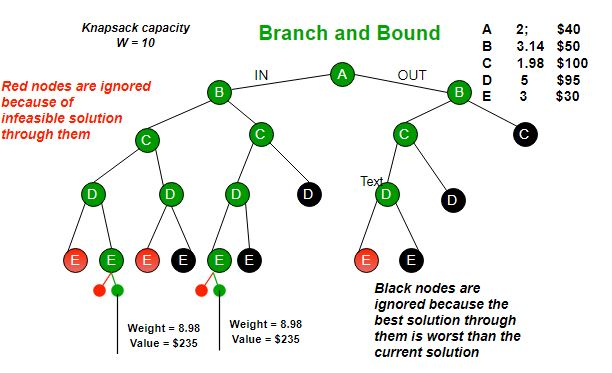Related Articles

# 0/1 Knapsack using Branch and Bound

• Difficulty Level : Medium
• Last Updated : 20 Nov, 2018

Branch and bound is an algorithm design paradigm which is generally used for solving combinatorial optimization problems. These problems typically exponential in terms of time complexity and may require exploring all possible permutations in worst case. Branch and Bound solve these problems relatively quickly.

Let us consider below 0/1 Knapsack problem to understand Branch and Bound.

Given two integer arrays val[0..n-1] and wt[0..n-1] that represent values and weights associated with n items respectively. Find out the maximum value subset of val[] such that sum of the weights of this subset is smaller than or equal to Knapsack capacity W.

Let us explore all approaches for this problem.

1. A Greedy approach is to pick the items in decreasing order of value per unit weight. The Greedy approach works only for fractional knapsack problem and may not produce correct result for 0/1 knapsack.
2. We can use Dynamic Programming (DP) for 0/1 Knapsack problem. In DP, we use a 2D table of size n x W. The DP Solution doesn’t work if item weights are not integers.
3. Since DP solution doesn’t alway work, a solution is to use Brute Force. With n items, there are 2n solutions to be generated, check each to see if they satisfy the constraint, save maximum solution that satisfies constraint. This solution can be expressed as tree.4. We can use Backtracking to optimize the Brute Force solution. In the tree representation, we can do DFS of tree. If we reach a point where a solution no longer is feasible, there is no need to continue exploring. In the given example, backtracking would be much more effective if we had even more items or a smaller knapsack capacity.Branch and Bound

The backtracking based solution works better than brute force by ignoring infeasible solutions. We can do better (than backtracking) if we know a bound on best possible solution subtree rooted with every node. If the best in subtree is worse than current best, we can simply ignore this node and its subtrees. So we compute bound (best solution) for every node and compare the bound with current best solution before exploring the node.

Example bounds used in below diagram are, A down can give \$315, B down can \$275, C down can \$225, D down can \$125 and E down can \$30. In the next article, we have discussed the process to get these bounds.Branch and bound is very useful technique for searching a solution but in worst case, we need to fully calculate the entire tree. At best, we only need to fully calculate one path through the tree and prune the rest of it.

Source:

Branch and Bound | Set 2 (Implementation of 0/1 Knapsack)

This article is contributed Utkarsh Trivedi. If you like GeeksforGeeks and would like to contribute, you can also write an article and mail your article to contribute@geeksforgeeks.org. See your article appearing on the GeeksforGeeks main page and help other Geeks.